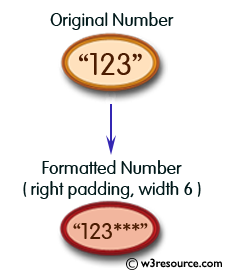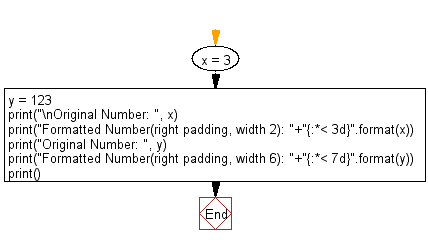﻿ Python: Print the following integers with '*' on the right of specified width - w3resource# Python: Print the following integers with '*' on the right of specified width

## Python String: Exercise-34 with Solution

Write a Python program to print the following integers with '*' on the right of specified width.Sample Solution:-

Python Code:

``````x = 3
y = 123
print("\nOriginal Number: ", x)
print("Formatted Number(right padding, width 2): "+"{:*< 3d}".format(x));
print("Original Number: ", y)
print("Formatted Number(right padding, width 6): "+"{:*< 7d}".format(y));
print()
```
```

Sample Output:

```Original Number:  3
Formatted Number(right padding, width 2): 3*
Original Number:  123
Formatted Number(right padding, width 6): 123***
```

Flowchart:## Visualize Python code execution:

The following tool visualize what the computer is doing step-by-step as it executes the said program:

Python Code Editor:

Have another way to solve this solution? Contribute your code (and comments) through Disqus.

What is the difficulty level of this exercise?

Test your Programming skills with w3resource's quiz.

﻿

## Python: Tips of the Day

What is the difference between Python's list methods append and extend?

append: Appends object at the end.

```x = [1, 2, 3]
x.append([4, 5])
print (x)
```

Output:

```[1, 2, 3, [4, 5]]
```

extend: Extends list by appending elements from the iterable.

```x = [1, 2, 3]
x.extend([4, 5])
print (x)
```

Output:

```[1, 2, 3, 4, 5]
```

Ref: https://bit.ly/2AZ6ZFq